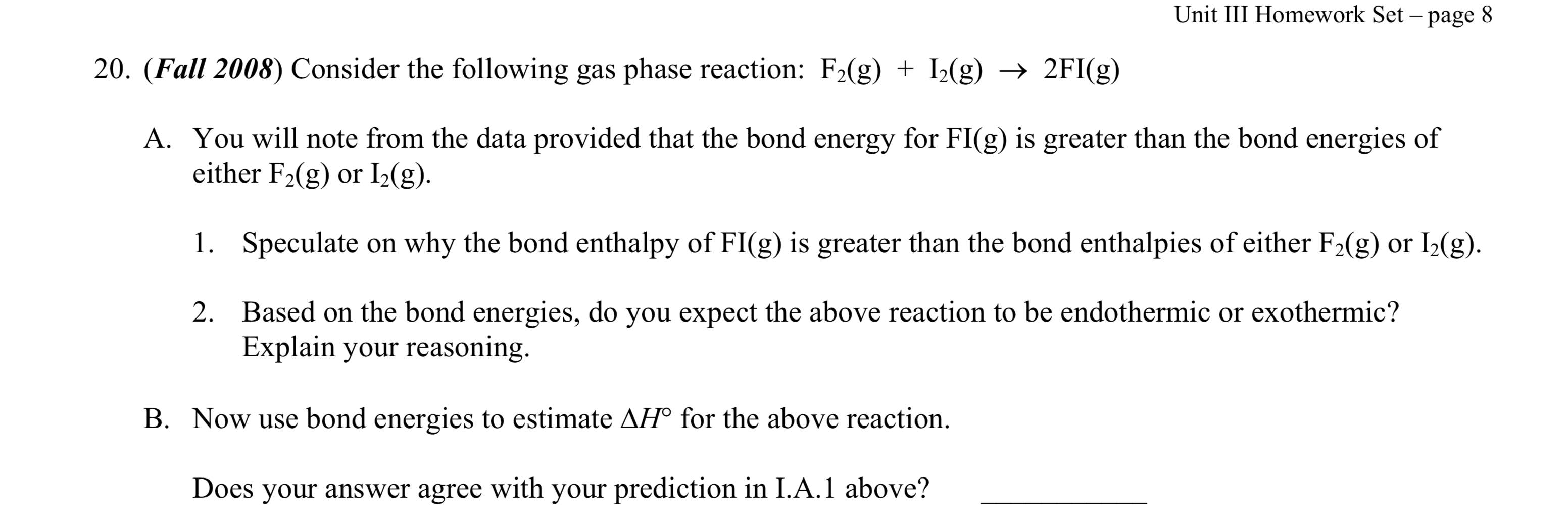# Consider the following gas phase reaction: F2(g) + I2(g) → 2 FI(g) You will note from the data provided that the bond energy for FI(g) is greater than the bond energies of either F2(g) or I2(g). 1. Speculate on why the bond enthalpy of FI(g) is greater than the bond enthalpies of either F2(g) or I2(g). 2. Based on the bond energies, do you expect the above reaction to be endothermic or exothermic? Explain your reasoning. B. Now use bond energies to estimate ΔH° for the above reaction. Does your answer agree with your prediction in I.A. 1 above?